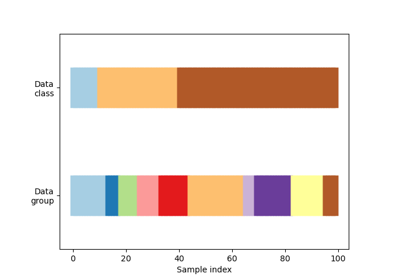# sklearn.model_selection.StratifiedGroupKFold¶

class sklearn.model_selection.StratifiedGroupKFold(n_splits=5, shuffle=False, random_state=None)[source]

Stratified K-Folds iterator variant with non-overlapping groups.

This cross-validation object is a variation of StratifiedKFold attempts to return stratified folds with non-overlapping groups. The folds are made by preserving the percentage of samples for each class.

Each group will appear exactly once in the test set across all folds (the number of distinct groups has to be at least equal to the number of folds).

The difference between GroupKFold and StratifiedGroupKFold is that the former attempts to create balanced folds such that the number of distinct groups is approximately the same in each fold, whereas StratifiedGroupKFold attempts to create folds which preserve the percentage of samples for each class as much as possible given the constraint of non-overlapping groups between splits.

Read more in the User Guide.

Parameters:
n_splitsint, default=5

Number of folds. Must be at least 2.

shufflebool, default=False

Whether to shuffle each class’s samples before splitting into batches. Note that the samples within each split will not be shuffled. This implementation can only shuffle groups that have approximately the same y distribution, no global shuffle will be performed.

random_stateint or RandomState instance, default=None

When shuffle is True, random_state affects the ordering of the indices, which controls the randomness of each fold for each class. Otherwise, leave random_state as None. Pass an int for reproducible output across multiple function calls. See Glossary.

StratifiedKFold

Takes class information into account to build folds which retain class distributions (for binary or multiclass classification tasks).

GroupKFold

K-fold iterator variant with non-overlapping groups.

Notes

The implementation is designed to:

• Mimic the behavior of StratifiedKFold as much as possible for trivial groups (e.g. when each group contains only one sample).

• Be invariant to class label: relabelling y = ["Happy", "Sad"] to y = [1, 0] should not change the indices generated.

• Stratify based on samples as much as possible while keeping non-overlapping groups constraint. That means that in some cases when there is a small number of groups containing a large number of samples the stratification will not be possible and the behavior will be close to GroupKFold.

Examples

>>> import numpy as np
>>> from sklearn.model_selection import StratifiedGroupKFold
>>> X = np.ones((17, 2))
>>> y = np.array([0, 0, 1, 1, 1, 1, 1, 1, 0, 0, 0, 0, 0, 0, 0, 0, 0])
>>> groups = np.array([1, 1, 2, 2, 3, 3, 3, 4, 5, 5, 5, 5, 6, 6, 7, 8, 8])
>>> sgkf = StratifiedGroupKFold(n_splits=3)
>>> sgkf.get_n_splits(X, y)
3
>>> print(sgkf)
StratifiedGroupKFold(n_splits=3, random_state=None, shuffle=False)
>>> for i, (train_index, test_index) in enumerate(sgkf.split(X, y, groups)):
...     print(f"Fold {i}:")
...     print(f"  Train: index={train_index}")
...     print(f"         group={groups[train_index]}")
...     print(f"  Test:  index={test_index}")
...     print(f"         group={groups[test_index]}")
Fold 0:
Train: index=[ 0  1  2  3  7  8  9 10 11 15 16]
group=[1 1 2 2 4 5 5 5 5 8 8]
Test:  index=[ 4  5  6 12 13 14]
group=[3 3 3 6 6 7]
Fold 1:
Train: index=[ 4  5  6  7  8  9 10 11 12 13 14]
group=[3 3 3 4 5 5 5 5 6 6 7]
Test:  index=[ 0  1  2  3 15 16]
group=[1 1 2 2 8 8]
Fold 2:
Train: index=[ 0  1  2  3  4  5  6 12 13 14 15 16]
group=[1 1 2 2 3 3 3 6 6 7 8 8]
Test:  index=[ 7  8  9 10 11]
group=[4 5 5 5 5]


Methods

 get_n_splits([X, y, groups]) Returns the number of splitting iterations in the cross-validator split(X[, y, groups]) Generate indices to split data into training and test set.
get_n_splits(X=None, y=None, groups=None)[source]

Returns the number of splitting iterations in the cross-validator

Parameters:
Xobject

Always ignored, exists for compatibility.

yobject

Always ignored, exists for compatibility.

groupsobject

Always ignored, exists for compatibility.

Returns:
n_splitsint

Returns the number of splitting iterations in the cross-validator.

split(X, y=None, groups=None)[source]

Generate indices to split data into training and test set.

Parameters:
Xarray-like of shape (n_samples, n_features)

Training data, where n_samples is the number of samples and n_features is the number of features.

yarray-like of shape (n_samples,), default=None

The target variable for supervised learning problems.

groupsarray-like of shape (n_samples,), default=None

Group labels for the samples used while splitting the dataset into train/test set.

Yields:
trainndarray

The training set indices for that split.

testndarray

The testing set indices for that split.

## Examples using sklearn.model_selection.StratifiedGroupKFold¶Visualizing cross-validation behavior in scikit-learn

Visualizing cross-validation behavior in scikit-learn